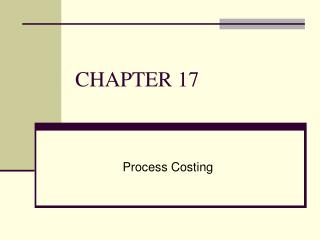# CHAPTER 17 - PowerPoint PPT PresentationDownload PresentationCHAPTER 17

CHAPTER 17Download Presentation## CHAPTER 17

- - - - - - - - - - - - - - - - - - - - - - - - - - - E N D - - - - - - - - - - - - - - - - - - - - - - - - - - -
##### Presentation Transcript

1. CHAPTER 17 Process Costing

2. Job-Costing and Process Costing: Opposite Ends of a Continuum Job-Costing Systems Distinct, identifiable units of a product or service Examples: Custom-made machines, Houses Process-Costing Systems Masses of identical or similar units of a product or service Examples: Food, Chemical processing

3. Process Costing • Process costing is a system where the unit cost of a product or service is obtained by assigning total costs to many identical or similar units • Each unit receives the same or similar amounts of direct materials costs, direct labor costs, and manufacturing overhead • Unit costs are computed by dividing total costs incurred by the number of units of output from the production process

4. Equivalent Units • A derived amount of output units that: • Takes the quantity of each input in units completed and in unfinished units of work in process and • converts the quantity of input into the amount of completed output units that could be produced with that quantity of input • Are calculated separately for each input (direct materials and conversion cost)

5. Example 1: Production Report – Weighted Average Method & FIFO Data for Dept.A: Costs info:

6. Example 2: …from Dept A to Dept B 31,000 units of output from Dept. A is transferred to Dept.B. Below are data for Dept.B:

7. Example 2 (continued) Costs info for Dept. B:

8. Abnormal Loss & Abnormal Gain Data: Input 1000 units @ RM4500 Normal loss 10% No opening or ending inventory. Required: Prepare Process Account in the following condition: • If actual output = 860 units, and total loss = 140 units. • If actual output = 920 units.

9. Abnormal Loss & Abnormal Gain In a period, there are 4 weeks. For week 3, input costs for a process was RM29,070 and other information were as follows: • Input 1000 units, Output 850 units,Normal loss 10% • As for week 4, input costs was also RM29,070, with input units of 1000 and output of 950 units. • There were no opening or closing inventory. Required: Prepare Process Accounts for week 3 and week 4.

10. Scrap, Abnormal Loss, Abnormal Gain A product undergone 2 processes: Process 1 and Process 2. Normal loss for each process is 10%. Scrap unit from Process 1 is sold at RM0.50 per unit and from Process 2 at RM3.00 per unit. Other relevant information are as follows: Actual OH is RM17,800. Required: (a) Process Account 1 & Process Account 2 (b) Scrap Account (c) Abnormal Loss Account & Abnormal Gain Account (d) Production OH Account

11. Ending Inventory ABC Sdn Bhd used process costing.In March 2005, there was no opening inventory but 5,000 units were put into production with the following costs: DM RM16,560; DL RM7,360; Prodn OH RM5,520. Out of those 5,000 input units, 4,000 units were completed during the month and transferred to the next process.The remaining 1,000 units of ending inventory were 60% completed with regards to materials and conversion. Required: Prepare Process Account.

12. Equivalent units & different stages of completion Info for Process 2 for the month of April 2005 are as follows: No opening WIP. Input from Process 1 4,000 units. Input costs: DM from Process 1 RM6,000; Additional DM in Process 2 RM1,080; Conversion costs RM1,720 Output were transferred to Process 3. Ending WIP was 800 units with the following stages of completion: DM from Process 1 100%; Addition DM 50%; Conversion costs 30%. Required: Prepare Process 2 Account for April 2005.

13. Changes in stock level and losses The following data is extracted from one process: No opening WIP Input 2,800 units Input costs RM16,695 Normal loss 10%, no scrap value Output 2,000 units WIP, ending 450 units, 70% completed Total loss 350 units Required: Prepare the Process Account.

14. Opening WIP There were 2 processes: Process 1 & 2. Info for Process 2 were: WIP, opening 800 units with completion stages: DM from Process 1 100%@RM4,700; Additional DM 40% @RM600; Conversion costs 30% @RM1,000. During the month, 3,000 units output from Process 1 were transferred to Process 2 valued at RM18,100. Additional DM costs in Process 2, RM9,600 and conversion costs RM11,800. Ending WIP 1,000 units (DM 100% completed referring to DM from Process 1 and 60% as for additional DM in Process 2 and 40% for conversion). Required: Prepare Process 2 Account using (a) Weighted Average Method (b) FIFO method.

15. Exercise Financial year for WWW Ltd ended 30th April. Process costing were used where products undergone 2 consecutive processes. Normal loss occurred in both processes at 10%. Relevant information:

16. During the month: OH was absorbed based on 150% of DL. Losses in Process 1 had no scrap value but in Process 2 can be sold at RM2 per kg.

17. Additional info: For both processes, ending WIP were 100% complete as for material but only 40% as for conversion. Ending finished goods inventory was valued at RM60,000 at the end of April 2005. Required: Prepare Process 1 and Process 2 Accounts for April 2005.